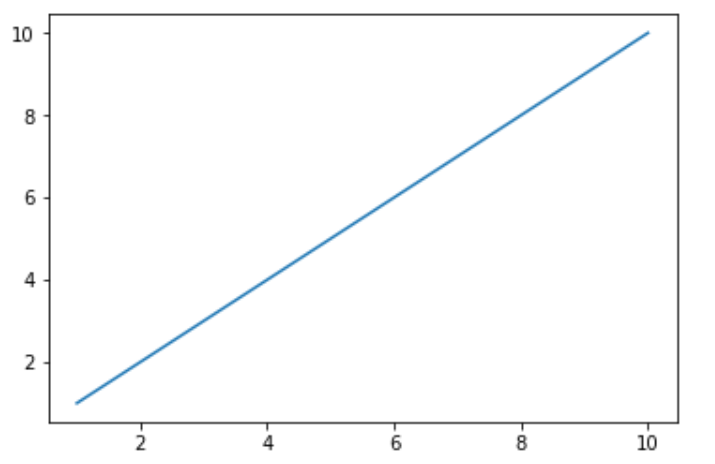# Python Pyforest Library

• Last Updated : 19 Feb, 2020

Sometimes, it happens that we spent a huge amount of time importing some common libraries like `NumPy`, `pandas`, `matplotlib`, `seaborn`, `nltk` and many more. To remove this headache of importing such libraries manually, we have `pyforest` library.

It is that library which helps you to work directly without importing other libraries separately.
It itself adds up some of the highly usable libraries used in DataScience while we are using it.

Attention geek! Strengthen your foundations with the Python Programming Foundation Course and learn the basics.

To begin with, your interview preparations Enhance your Data Structures concepts with the Python DS Course. And to begin with your Machine Learning Journey, join the Machine Learning - Basic Level Course

Functions of pyforest :

• active_imports(): It will return all the libraries which have been used in the program.
• lazy_imports(): It will return all the libraries available in pyforest.

Installing Library:

`pip install pyforest`

Let’s see the usage of `pyforest` with various libraries.

• Numpy: NumPy is a general-purpose array-processing package. It provides a high-performance multidimensional array object, and tools for working with these arrays.

Example:

 `# here we have not import  ``# 'numpy as np' by explicitly ``a ``=` `np.array([[``1``, ``2``, ``3``], [``4``, ``5``, ``6``], [``7``, ``8``, ``9``]]) `` ` `print``(a)`

Output:

```[[1 2 3]
[4 5 6]
[7 8 9]]
```

• Pandas: Pandas DataFrame is two-dimensional size-mutable, potentially heterogeneous tabular data structure with labeled axes (rows and columns). A Data frame is a two-dimensional data structure, i.e., data is aligned in a tabular fashion in rows and columns. Pandas DataFrame consists of three principal components, the data, rows, and columns.

Example:

 `d ``=` `{``'A'``:[``1``, ``2``, ``3``], ``'B'``:[``4``, ``5``, ``6``], ``'C'``:[``7``, ``8``, ``9``]}`` ` `# here we have not import``# 'pandas as pd' by ourself .``df ``=` `pd.DataFrame(d)  `` ` `print``(df)`

Output:

```   A  B  C
0  1  4  7
1  2  5  8
2  3  6  9
```

• NLTK: The NLTK module is a massive tool kit, aimed at helping you with the entire Natural Language Processing (NLP) methodology.

Example:

 `# here we do not import``# ' Nltk library' by ourself``# but only the class of nltk .``from` `nltk.tokenize ``import` `word_tokenize`` ` `data ``=` `"All apples are red in colour"`` ` `print``(word_tokenize(data))`

Output:

`['All', 'apples', 'are', 'red', 'in', 'colour']`

Note: For more information, refer to Tokenize text using NLTK in python

• Matplotlib: Matplotlib is an amazing visualization library in Python for 2D plots of arrays. Matplotlib is a multi-platform data visualization library built on NumPy arrays and designed to work with the broader SciPy stack.

Example:

 `# here we have not imported ``# 'matplotlib.pyplot as plt' by ourself.`` ` `x ``=` `[``1``, ``2``, ``3``, ``4``, ``5``, ``6``, ``7``, ``8``, ``9``, ``10``]``y ``=` `[``1``, ``2``, ``3``, ``4``, ``5``, ``6``, ``7``, ``8``, ``9``, ``10``] `` ` `plt.plot(x, y)  ``plt.show()`

Output: